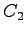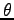# Convergence3

Convergence for Eigenvalue Problems with Mesh Adaptivity

Convergence result:

The main result of this paper is Theorem 3.2 below which proves convergence of the adaptive method and also demonstrates the decay of oscillations of the sequence of approximate eigenfunctions. Before proving this result we need a final lemma.

Theorem 3.2 Provided the initial mesh is chosen so thatis small enough, there exists a constant such that the recursive application of Algorithmyields a convergent sequence of approximate eigenvalues and eigenvectors, with the property:

where andare positive constants.
The initial mesh convergence threshold and the constantsandmay depend on,and.